Standardization of NaOH and Percentage Error Report

Preparation of a Standard Base and Analysis of an Unknown

Preliminary Results 1:

Molare mass of KHP = 204.23g/mol

Trial # 1:

Amount of KHP = 0.875g

0.875g x x = 0.0043mol of NaOH

Volume of NaOH = 42.8mL x = 0.0428L

[NaOH] = = = 0.1001M

Trial # 2:

Amount of KHP = 0.792g

0.792g x x = 0.0039mol of NaOH

Volume of NaOH = 40.6mL x = 0.0406L

[NaOH] = = = 0.0955M

Trial # 3:

Amount of KHP = 0.820g

0.820g x x = 0.0040mol of NaOH

Volume of NaOH = 39.1mL x = 0.0391L

[NaOH] = = = 0.1027M

Mean of [NaOH] = = 0.0994M

Preliminary Results 2:

Data:

 Flask # Amount of KHP (g) Volume of NaOH (mL) 1 1.2864 32.34 2 1.3119 33.41 3 1.5801 40.55

Molar mass of KHP = 204.23g/mol

[NaOH] = 0.09943M

Calculations:

32.34mL of NaOH x xx x= 0.6565g

WT% = x100% = 51.04%

 Flask # Calculated mass of KHP (g) WT% 1 0.6565 51.04 2 0.6784 51.71 3 0.8234 52.11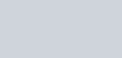EXPERIMENT 2; PREPARATION OF A STANDARD BASE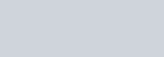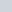AND ANALYSIS OF AN UNKNOWNSodium hydroxide,NaOH is a sfrong base commonly used in titrations. However, NaOH solutions also often contain the weak base bicarbonate (HC03-) due to reaction with atmospheric carbon dioxide (C02).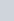# OH- + C02 HC03′Therefore, we must determine the real concentration ofNaOH in solution by titration with a primary standard before using the NaOH to titrate an unknown. Primary standards are reagents that are pure and stable enough to be used directly after weighing. In this experiment, we will be using potassium hydrogen phthalate (KHP, KHC8H404) as our primary standard to determine the exact concentration ofNaOH. Then, we will use our standardized NaOH to determine the amount of KHP in an unknown sample.

Chemicals/Reagents and additional materials required:

e —0.1M sodium hydroxide (NaOH) solution (2L per student, same as Exp, l)

• Phenolphthalein indicator solution (l dropper bottle per student)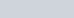• Potassium hydrogen phthalate (KHP, KHC8H404, 204.23 g/mol) (—5g per student,to be dried)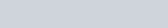•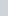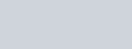Unknown sample containing potassium hydrogen phthalate (KHP) (—5g perstudent, to be dried)## I. EXPERIMENTALA. Preparation of standard and unknown KHP:

1. Dry —5g of standard potassium hydrogen phthalate (KHP, KHC8H404) at 110 OCTor 1 hr. Cool standard KHP in a desiccator.2. Dry your entire portion of sample containing an unknown amount ofKHP at 110 oc for 1 hr. Cool unknown KHP sample in a desiccator.

B. Standardization ofNaOH

le The NaOH solution provided is —0.1M. Calculate Of I the M amount according of dried to the

reaction below:

I C H 04 KHC8H404 + NaOH KNaC8H404 + 1-120

NqcK 2. Accurately weigh 3 samples (by difference) of dry standard KHP (within10% of the previously calculated value in step I.B.I above) into 3 separate250 mL Erlenmeyer flasks.3. Dissolve each standardo J 927bKHP

sample

in

50

100

mL

of

deionized

water.

4.

Titrate

with

NaOH

solution

72M

o,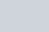C. Analysis ofI.needed to require ofNaOH for complete titration,1. Accurately wergh samples (by difference) of unknown sample containingKHP (within 10% of the previously calculated value in step l.c. I. above) intoø 3 separate 250 mL Erlenmeyer flasks.1.Dissolve each unknown sample containing K-HP in 50-100 mL of deionized2. Titrate with NaOH solution to a phenolphthalein end point.## Il. PRELIMINARY RESULTS

1. From your results in I.B (Standardization ofNaOH), calculate the real [NaOHl using each of your standard KHP titration trials. Then, calculate a mean [NaOHl.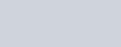2. From your results in l.c (Analysis ofan Unknown containing KHP), calculate the weight percent of KHP in your unknown sample usmg each of your unknown KHP titration trials. Then, calculate a mean wt% KHP.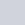### 111. RESULTS (These will build up on the Preliminary Results in part Il above)

1.From your results in I.B (Standardization ofNaOH), calculate the real [NaOH] using each of your standard KHP titration trials. Then, calculate amean, standard deviation, and 95% confidence interval for the [NaOH].

1. From your results in l.c (Analysis ofan Unknown containing KHP), calculate the weight percent ofKHP in your unknown sample using each of your unknown KHP titration trials. Then, calculate a mean, standard deviation, and 95% confidence interval for the wt% KHP.
2.Email your instructor with the label of your KHP unknown — she will provide you with the actual wt% KHP in your fitnknown” sample.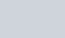. D so
1. Is your standard [NaOHl accurate and/or precise? How do you know? What could be done differently to improve results?
2.yourunknown accurate and/or precise? How do you know? What could be done differently to improve results?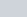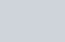0. ICdN) o. 16

1 q 09 €6

Preparation of a Standard Base and Analysis of an Unknown

Preliminary Results:

Data:

 Flask # Amount of KHP (g) Volume of NaOH (mL) 1 1.2864 32.34 2 1.3119 33.41 3 1.5801 40.55

Molar mass of KHP = 204.23g/mol

[NaOH] = 0.09943M

Calculations: